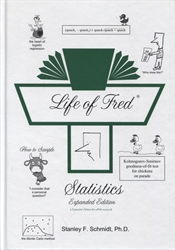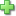# Life of Fred: Statistics (Expanded Edition)

## (as serious as it needs to be)

by Stanley F. Schmidt
Hardcover, 576 pages
Price: \$57.00

Statistics is the second book in the Life of Fred University Mathematics Series, however, it can be used before calculus if desired; it provides one years' worth of college-level statistics. There is one half-chapter that does require calculus, but is not necessary to complete the course. Students should have basic knowledge of algebra; a very brief 5-question placement exam is provided at the beginning to test student familiarity with basic concepts.

Eight chapters are included as well as a "field guide." Within each chapter "Your Turn to Play" sections are provided, which give students the opportunity to practice new concepts with problems (and have completely worked-out solutions to check answers!) Six "cities" exercises are featured at the end of each chapter; sets are named after a city and should each take approximately 20-40 minutes to complete. Answers to all "cities" problems are included in this "expanded edition."

The Field Guide is designed for students who need to understand statistics quickly; they can simply turn to the guide and learn what test to use, where to find an explanation of the test, where it's found in the book, and what table to use.

Life of Fred Statistics covers: Descriptive Statistics (averages, measures of dispersion, types of distributions), Probability, Bayes' Theorem, From a Given Population Determine What Samples Will Look Like (7 tests), Techniques of Sampling, From a Given Sample Determine What the Population Was (14 tests), Determine Whether Two Given Samples Came From the Same Population (15 tests), Working With Three or More Samples (10 tests), Emergency Statistics Guide, and Regression Equations.

• All the popular tests! Normal Distribution, Chi-Squared, etc.
• Emergency Statistics Guide!
• Field Guide!

Descriptive Statistics (averages, measures of dispersion, types of distributions), Probability, Bayes' Theorem, From a Given a Population Determine What Samples Will Look Like (7 tests), Techniques of Sampling, From a Given Sample Determine What the Population Was (14 tests), Determine Whether Two Given Samples Came From the Same Population (15 tests), Working With Three or More Samples (10 tests), Emergency Statistics Guide, Regression Equations, Field Guide, 16 Tables.

This expanded edition includes all answers! Do not order the answer key with this book.

Did you find this review helpful?
Series Description
Related CategoriesClick here to write a review